Trial ends in

A subscription to JoVE is required to view this content.
You will only be able to see the first 20 seconds.

Friction
Science Education (Physics I)
Kinematics and Projectile Motion
Entropy

Friction

Overview

Source: Nicholas Timmons, Asantha Cooray, PhD, Department of Physics & Astronomy, School of Physical Sciences, University of California, Irvine, CA

The goal of this experiment is to examine the physical nature of the two types of friction (i.e., static and kinetic). The procedure will include measuring the coefficients of friction for objects sliding horizontally as well as down an inclined plane.

Friction is not completely understood, but it is experimentally determined to be proportional to the normal force exerted on an object. If a microscope zooms in on two surfaces that are in contact, it would reveal that their surfaces are very rough on a small scale. This prevents the surfaces from easily sliding past one another. Combining the effect of rough surfaces with the electric forces between the atoms in the materials may account for the frictional force.

There are two types of friction. Static friction is present when an object is not moving and some force is required to get that object in motion. Kinetic friction is present when an object is already moving but slows down due to the friction between the sliding surfaces.

Principles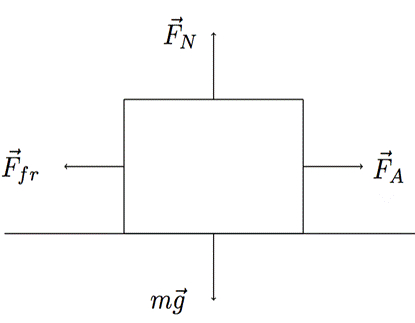Figure 1.

Figure 1 shows four forces acting on an object that sits on a horizontal plane.corresponds to some applied horizontal force.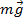is the force of gravity on the object, which is matched equally but in the opposite direction by the normal force,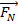. The normal force is a result of a surface acting on an object in opposition to gravity. The normal force explains why a book does not simply fall through the table it is resting upon. Finally, opposing the applied force is the frictional force,. The frictional force is proportional to the normal force: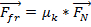, (Equation 1)

where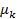is the coefficient of friction.

The coefficient of friction must be measured experimentally and is a property that depends upon the two materials that are in contact. There are two types of coefficients of friction: kinetic friction,, when objects are already in motion, and static friction,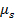, when objects are at rest and require a certain amount of force to get moving. For an object sliding along a path, the normal force is equal to the weight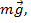of the object. Therefore, the frictional force depends only upon the coefficient and the mass of an object.

If the object is on an inclined plane, then the normal forceis perpendicular to the incline and is not equal and opposite to the weightas can be seen in Figure 2.Figure 2.

In this case, only a component ofis equivalent to the normal force, depending on the angle θ: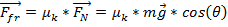. (Equation 2)

The angle of repose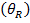is defined as the point at which the force of gravity on an object overcomes the static friction force and the object begins to slide down an inclined plane. A good approximation for the angle of repose is: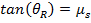. (Equation 3)

In this lab, two metal pans will be used to represent materials with different coefficients of friction. Block A will have a sand paper bottom, which will result in a higher coefficient of friction, while block B will have a smooth metal bottom.

Procedure

1. Measure the coefficients of friction.

1. Add a 1,000-g weight to each block and use a scale measure the masses of blocks A and B, including the added mass.
2. Connect the force scale to block A. Pull the scale horizontally and note the reading just before the block begins to slide. Just before it begins to slide, the maximum amount of static friction is resisting the movement. Use the force reading to calculatefor block A. Do this five times and record the average value.
3. Repeat step 1.2 with block B.
4. Pull block A across the table at a constant speed. If the speed is constant, then the force reading on the scale should be equal to the frictional force. Calculatefor block A. Do this five times and record the average value.
5. Repeat step 1.4 with block B.

2. Effect of weight on the force of friction.

1. Place block A on top of block B and repeat step 1.4 five times, determining the average value. Calculate the factor by which the frictional force increased/decreased.
2. Place block B on top of block A and repeat step 1.4 five times, determining the average value. Calculate the factor by which the frictional force increased/decreased.

3. Effect of surface area on force of friction.

1. Turn block B onto the side that contains only the rim of the pan. The weight will need to be placed on the top of the face-up side. Measure the force of friction and compare it to the value measured in step 1.2. Calculate the factor by which the frictional force increased/decreased.

4. Angle of repose.

1. Place block A on the adjustable incline plane, starting at an angle of 0°. Slowly raise the angle until the block begins to slide. Using a protractor, measure the angle of repose and use Equation 3 to calculate the coefficient of static friction just before the block began to slide. Do this five times and record the average value.
2. Repeat step 4.2 with block B.

The effects of friction are easily observed in everyday activities and yet the physical mechanisms that govern friction can be complex.

Friction is a force that opposes the motion of an object when it is in contact with a surface. At the microscopic level, it is caused by surface roughness of the materials in contact and intermolecular interactions. But one can overcome this force by application of an external force that is equal in magnitude.

The goal of this video is to demonstrate how to measure friction in a lab setting for objects sliding horizontally as well as down an inclined plane.

Before diving into the protocol, let's revisit the concepts behind the frictional force. First, you need to know that there are two types of frictions - kinetic friction and static friction.

To understand kinetic friction, imagine you are in a rubber tube sliding across an infinite horizontal field of ice.

Although ice may be considered a smooth surface, if we look at the microscopic level, there are complex interactions between the two surfaces that cause friction. These interactions depend on surface roughness and attractive intermolecular forces.

The magnitude of this kinetic friction force is equal to the product of the coefficient of kinetic friction, or μK, which depends on the material-surface combination, and the normal force, or Fnorm that pushes the object and surface together.

Fnorm acts to support the object and is perpendicular to the interface. In this case, since the tube is on a level ground, the Fnorm is equal to and opposite the force of gravity, which is mg. Therefore, if you know the combined mass of you with the tube, and the coefficient of kinetic friction for rubber and ice, we can easily calculate the force of friction.

Kinetic friction can convert some of the tube's kinetic energy into heat and will also reduce the momentum of the tube ultimately bringing it to rest.

Now, this is when static friction - the other type of friction - comes into play. This frictional force opposes movement of a static object and could be calculated by applying an external force. The applied force that eventually moves the object reveals the maximum static force.

The formula for maximum static force is the same as the one for kinetic friction, but the coefficient of static friction μS is typically greater than μK for the same material-surface combination.

Another way to overcome the maximum static force is by increasing the slope of the surface. At some angle, called the angle of repose or θR, the force pulling down the slope will equal the static friction force and the tube will begin to slide. This pulling force, which is the sine of the angle of repose times the force of gravity, equals the maximum static force, which is μS times product of m, g, and cosine of θR. By rearranging this equation, we can calculate the coefficient of static friction.

Now that we've learned the principles of friction, let's see how these concepts can be applied to experimentally calculate the forces and coefficients of both kinetic and static friction. This experiment consists of a mass scale, a force scale, two metal pans with different coefficients of friction denoted as block 1 and 2, an adjustable incline plane, two 1000 g weights, and a protractor.

Add a 1000 g weight to each block and use the scale to measure the masses of the loaded blocks.

After connecting the force scale to block 1, pull the scale horizontally and note the force reading just before the block begins to slide. Record this maximal static friction force and repeat this measurement five times to obtain multiple data sets. Perform the same procedure using block 2 and record these values.

Next, with the force scale connected to block 1, pull the scale at a constant speed and note the kinetic friction force on the gauge. Repeat this measurement five times to obtain multiple data sets. Again, perform the same procedure using block 2 and record these values.

Now, place block 1 on top of block 2 and pull the scale at a constant speed to determine the kinetic friction force. Repeat this measurement five times and calculate the average. Then perform the same procedure with block 2 on top of block 1.

For the next experiment, turn block 1 such that the smaller surface area faces the table and attach it to the force scale. Now measure the static friction force as before by making note of the force before the block begins to slide. Repeat this measurement five times to obtain multiple data sets.

For the last experiment, place block 1 on the adjustable incline plane with the plane initially at an angle of zero degrees. Slowly raise the angle of the plane and use a protractor to determine the angle at which the block begins to slide. Again, repeat this measurement five times to obtain multiple data sets and perform the same procedure using block 2.

For the experiments performed on horizontal surface, the normal force on the blocks is equal to the weight, that is mass times g. Since the mass of block 1 and 2 for both static and kinetic friction experiments are the same, Fnorm is the same in all four cases. Using the average of the measured force values for the various experiments, and the formulae for both frictions, the coefficients of friction can be calculated.

As expected, the coefficient of static friction is greater than the coefficient of kinetic friction. Furthermore, the respective coefficients for the two blocks are different since they each possess a different surface roughness.

In the stacked blocks experiment, we know that the mass doubles in both cases, so we can calculate the new Fnorm. We already know μk for the block in contact with the surface. Using this we can calculate the kinetic friction force, which agrees well with the measured force during the experiment.

The friction force measured following a change in orientation of block 1 demonstrated that the contact surface area does not affect the force of friction. The discrepancies between the calculated and measured forces are consistent with the estimated errors associated with reading the force scale while maintaining a constant speed.

For the inclined plane experiments, the angle of repose was measured. Using this angle, the coefficients of static friction could be determined, and here the values compare favorably with the coefficients measured from the horizontal sliding measurements.

Studying friction is important in several applications, as it can either be highly beneficial or a phenomenon that must be minimized.

It is extremely important for automobile tire manufactures to study friction, as it allows tires to gain traction on a road. Therefore, when it rains, the water and residual oils on the road significantly reduce the coefficient of friction, making sliding and accidents much more likely.

While engineers want to increase friction for car tires, for engines and machinery in general they want to reduce it, as friction between metals can generate heat and damage their structures. Therefore, engineers constantly study lubricants that may help in decreasing the coefficient of friction between two surfaces.

You've just watched JoVE's introduction to Friction. You should now understand what factors contribute to the magnitude of friction, the different types of friction, and the underlying physical mechanisms that govern it. As always, thanks for watching!

Results

Table 1. Coefficients of friction.

 BlockA 0.68 0.60 B 0.52 0.47

Table 2. Effect of weight and surface area on the force of friction.

 Measurement(N) Factor by which it is larger or smaller Block B on A 16 Withfrom step 1.4 = 2.3 Block A on B 14 Withfrom step 1.5 = 2.5 Small surface area 5 Withfrom step 1.4 = 0.9

Table 3. Angle of repose.

 Block Angle of repose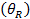(°)A 30 0.58 B 24 0.45

The results obtained from the experiment match the predictions made by Equations 1 and 2. In step 1, the static friction was larger than the kinetic friction. This is always the case, as more force is required to overcome friction when an object is not already in motion. In step 2, it was confirmed that the force of friction was proportional to the weight of both blocks and the coefficient of kinetic friction of the block in contact with the table. The result of step 3 confirms that the surface area does not affect the force of friction. In step 4, the angle of repose can be approximated by Equation 3. The error associated with the lab comes from the difficulty of reading the force scale while maintaining a constant velocity for the sliding block. By taking several measurements and calculating the average, this effect can be reduced.

Applications and Summary

Friction is everywhere in our daily lives. In fact, it would not be possible to walk without it. If someone tried walking on a frictionless surface, he would go nowhere. It is only the friction between the bottom of his feet and the ground as his muscles push against the ground that propels him forward.

In almost every aspect of industry, engineers are trying to reduce friction. When two surfaces are in contact, there will always be friction. This can take the form of heat, such as the heat felt when someone quickly rubs her hands together. In industrial applications, this heat can damage machines. Friction forces also oppose the motion of objects and can slow done mechanical operations. Therefore, substances like lubricants are used to decrease the coefficient of friction between two surfaces.

Table 4. Example coefficients of friction.

 Materialswood on wood 0.2 brass on steel 0.44 rubber on concrete 0.8 lubricated ball bearings < 0.01

In this experiment, the coefficients of static and kinetic friction were measured for two different sliding blocks. The effect of mass on the force of friction was examined, along with the effect of surface area. Lastly, the angle of repose for a block on an inclined plane was measured.X